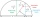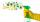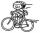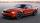# HP - harmonic progression

Determine the 10th term of the harmonic progression 6,4,3,…

h10 =  1.0909

### Step-by-step explanation:Did you find an error or inaccuracy? Feel free to write us. Thank you!Tips to related online calculators
Looking for calculator of harmonic mean?
Looking for a statistical calculator?

## Related math problems and questions:

• HP - harmonic progressionDetermine the 8th term of the harmonic progression 2, 4/3, 1,…
• HP - harmonic progression 2Compute the 16th term of the HP if the 6th and 11th term of the harmonic progression are 10 and 18 respectively.
• Harmonic meanIf x, y, z form a harmonic progression, then y is the harmonic mean of x and z. Find the harmonic mean of the numbers 6 and 5.
• Sequence 11What is the nth term of this sequence 1, 1/2, 1/3, 1/4, 1/5?
• Arithmetic progression 2The 3rd term of an Arithmetic progression is ten more than the first term, while the fifth term is 15 more than the second term. Find the sum of the 8th and 15th terms of the Arithmetic progression if the 7th term is seven times the first term.
• Insert 5Insert five harmonic means between 1/2 and 1/26
• Insert 6Insert four harmonic means between 3/7 and 3/19
• Insert 7Insert five harmonic means between 3 and 18
• Harmonic meanHarmonic means of 6 and 12
• Harmonic 4The harmonic mean of -6 and 5.
• 10th termWhat is the 10th term of the Arithmetic Progression if x1=4 and d=5?
• CleanersMilan would clean up the room for 2.5 hours, Eric would take 10 hours. How long they swept the room together?
• Weighted harmonic averageTen workers will do some work in 2 minutes, five workers in 10 minutes and three workers in 6 minutes. How many minutes per average worker per worker?
• PoolIf water flows into the pool by two inlets, fill the whole for 19 hours. The first inlet filled pool 5 hour longer than the second. How long pool take to fill with two inlets separately?
• What is 10What is the 5th term, if the 8th term is 80 and common ratio r =1/2?
• CyclistThe cyclist goes uphill 10 km for 50 minutes and downhill minutes for 29 minutes, both applied to the pedals the same force. How long he pass 10 km by plane?
• CarThe car goes from point A to point B at speed 86 km/h and back 53 km/h. If it goes there and back at speed 67 km/h trip would take 10 minutes shorter. What is the distance between points A and B?